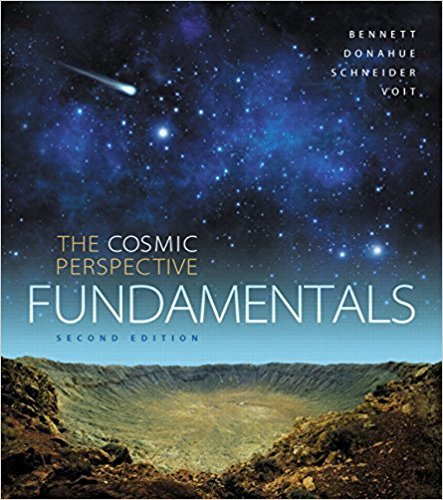×
Get Full Access to The Cosmic Perspective Fundamentals - 2 Edition - Chapter 7 - Problem 19seq
Get Full Access to The Cosmic Perspective Fundamentals - 2 Edition - Chapter 7 - Problem 19seq

×

# Explain answer clearly, with complete | Ch 7 - 19SEQISBN: 9780133889567 46

## Solution for problem 19SEQ Chapter 7

The Cosmic Perspective Fundamentals | 2nd Edition

• Textbook Solutions
• 2901 Step-by-step solutions solved by professors and subject experts
• Get 24/7 help from StudySoup virtual teaching assistantsThe Cosmic Perspective Fundamentals | 2nd Edition

4 5 1 235 Reviews
27
0
Problem 19SEQ

Problem 19SEQ

Explain answer clearly, with complete sentences and proper essay structure if needed. An asterisk (*) designates a quantitative problem, for which you should show your work.

*Planet Around 51 Pegasi. The star 51 Pegasi has about the same mass as our Sun. A planet discovered around it has an orbital period of 4.23 days. The mass of the planet is estimated to be 0.6 times the mass of Jupiter. Use Kepler’s third law to find the planet’s average distance (semimajor axis) from its star. (Hint: Because the mass of 51 Pegasi is about the same as the mass of our Sun, you can use Kepler’s third law in its original form, p2 = a3. Be sure to convert the period into years before using this equation.)

Step-by-Step Solution:

Step 1 of 2

We need to find the average distance (semimajor axis) of a planet which is orbiting the star 51 Pegasi.

The planet’s distance (semimajor axis) from its star can be found using Kepler’s third law which states that “ the square of orbital period of a planet is directly proportional to the cube of the semi- major axis of its orbit”.Where,orbital period of the planet in Earth yearsaverage distance of the planet from the Sun in AU

Step 2 of 2

##### ISBN: 9780133889567

The full step-by-step solution to problem: 19SEQ from chapter: 7 was answered by , our top Physics solution expert on 07/18/17, 06:37AM. This textbook survival guide was created for the textbook: The Cosmic Perspective Fundamentals, edition: 2. The answer to “Explain answer clearly, with complete sentences and proper essay structure if needed. An asterisk (*) designates a quantitative problem, for which you should show your work.*Planet Around 51 Pegasi. The star 51 Pegasi has about the same mass as our Sun. A planet discovered around it has an orbital period of 4.23 days. The mass of the planet is estimated to be 0.6 times the mass of Jupiter. Use Kepler’s third law to find the planet’s average distance (semimajor axis) from its star. (Hint: Because the mass of 51 Pegasi is about the same as the mass of our Sun, you can use Kepler’s third law in its original form, p2 = a3. Be sure to convert the period into years before using this equation.)” is broken down into a number of easy to follow steps, and 125 words. This full solution covers the following key subjects: mass, planet, pegasi, period, star. This expansive textbook survival guide covers 15 chapters, and 313 solutions. Since the solution to 19SEQ from 7 chapter was answered, more than 403 students have viewed the full step-by-step answer. The Cosmic Perspective Fundamentals was written by and is associated to the ISBN: 9780133889567.

Unlock Textbook Solution